# Daphmacromine A Structure Elucidation

### Daphmacromine A

Daphniphyllum alkaloids are a family of structurally diverse natural products with complex polycyclic systems elaborated by plants of the genus Daphniphyllum. They are characterized by unique structural features which have attracted great interest of researchers. M. Cao and co-workers  recently described the isolation and structure elucidation of 10 new alkaloids belonging to this family. The spectral data presented for one of the compounds, Daphmacromine A (C25H37NO5, Structure 1), were used to challenge ACD/Structure Elucidator.

The 1H and 13C chemical shifts of this compound were
presented by authors in a table , but the COSY and key HMBC correlations were depicted graphically as shown below: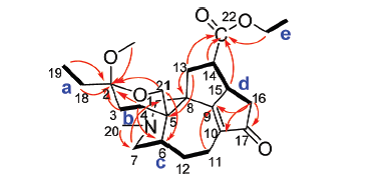1H-1H COSY (bold) and key HMBC correlations of Structure 1

1H-1H COSY (bold) and key HMBC correlations of Structure 1

Despite the absence of a complete HMBC data set, we decided to attempt to elucidate this structure with Structure Elucidator. The molecular formula and available 1D and 2D NMR data were input into the program, which created the Molecular Connectivity Diagram (MCD):

Using systems knowledge of spectrum-structure correlations, the program automatically determined atom hybridizations (blue atoms: sp3; violet atoms: sp2; light blue atoms: sp3 or sp2) and the possibility of a heteroatom as a neighbor (“ob”—obligatory, “fb”—forbidden). Note that hybridization of atom C(98.90) is marked as either sp3 or sp2, because the possibilities of both C=C and O-C-C atomic environments are taken into account.

At the same time, atom C(209.20) has been labeled “ob” (neighborhood heteroatom is obligatory), while atoms C(173.20) and C(183.70) have no labels indicating the presence (ob) or absence (fb) of a heteroatom in the first sphere of the atom environment. Generally, when the molecule in question belongs to a common (trivial) chemical compound, we assume that the spectroscopist would assign C(173.20) as an ester or amide, and C(183.70) as either one of latter or as a ketone. But it is seen from Structure 1 that atom C(183.70) is part of a C=C double bond and has neither O or N in its closest environment. It turns out that this chemical shift value is intrinsic for all Daphniphyllum alkaloids.

Because we are simulating a real situation where Structure Elucidator is utilized to elucidate a completely unknown structure, we initially investigated what solution would be obtained if we only relied on “common sense” and ascribed the label “ob” to both carbon atoms C(173.20) and C(183.70). Note that by adding these labels, we introduce new structural constraints, which in turn reduces the number of possible structures and time of structure generation. Therefore the properties of the C(173.20) and C(183.70) atoms were modified on the MCD, and structure generation was initiated without further changes to the standard options.

Results: k=2816→2320→1056 (2816 structures generated, reduced to 1056 after removal of duplicates); generation time tg = 11s.

After 13C chemical shift prediction and structure ranking according to standard methodology common for the Structure Elucidator system, a ranked output of structures was obtained. The four top structures of the ranked file along with chemical shift deviations calculated are shown below: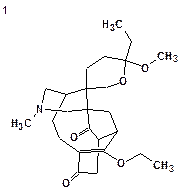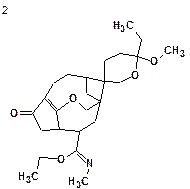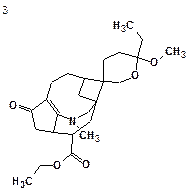dA = 4.260 (v12.01) dA = 4.794 (v12.01) dA = 4.367 (v12.01) dA = 5.388 (v12.01) dI = 4.493 dI = 4.496 dI = 4.602 dI = 4.712 dN = 3.938 dN = 5.096 dN = 4.663 dN = 5.041 max_dA = 24.320 max_dA = 19.020 max_dA = 15.440 max_dA = 28.220 max_dI = 20.997 max_dI = 17.792 max_dI = 16.777 max_dI = 17.852 max_dN = 22.565 max_dN = 21.270 max_dN = 20.452 max_dN = 25.231

We see that both average and maximal deviations are higher than typically acceptable for the first (“best”) structure, and consequently the structural constraints introduced by us were mistaken. Note that within several minutes we were able to reject the initial assumptions as incorrect.

The second run was performed from the initial MCD that was automatically created by the program.

Result: k = 7822→6542→2255, tg=32 s.

Removing constraints increased the size of the output file (double the number of proposed structures) and time of structure generation (three times longer but still less than a minute).

The top structures of the ranked file are shown below: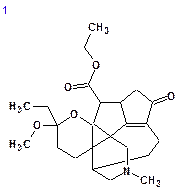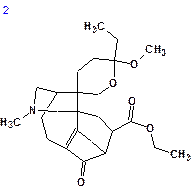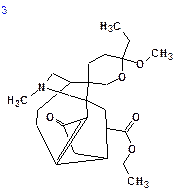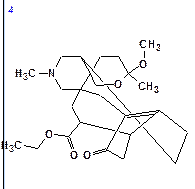dN = 2.105 dN = 2.579 dN = 3.019 dN = 3.290 dI = 2.311 dI = 2.681 dI = 3.621 dI = 3.479 dA = 2.223 dA = 2.556 dA = 3.400 dA = 3.131

We see that the best structure coincides with Structure 1 and the chemical shift deviations are similar to the values commonly observed for problems successfully solved using Structure Elucidator.

This example shows that even well known spectrum-structure correlations can be violated in complex polycyclic structures. However utilizing Structure Elucidator allows researchers to minimize the amount of expert assumptions (“axioms”) necessary and get the correct solution to the problem in a short time. Where common assumptions are made by the researcher, the program makes it easy to recognize when these are incorrect.

### References

1. Daphmacromines A-J, Alkaloids from Daphniphyllum macropodum, Mingming Cao, Yu Zhang, Hongping He, Shifei Li, Shengdian Huang, Duozhi Chen, Guihua Tang, Shunlin Li, Yingtong Di, Xiaojiang Hao, J. Nat. Prod., 75(6):1076–1082, 2012.